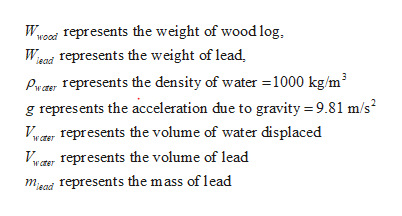# The density of a floating object can be determined by tying just enough weight to the object to submerge it completely, and then weighing the weights and object separately in the air. Consider a wood log that weights 1600 N in air. It is found that it takes 42 kg of lead (????? = 11,300 ?? ?3 ⁄ ) to just submerge the log in the water (i.e. to achieve neutral buoyancy). For the conditions given, find thefollowing:a. The volume of the log in m3.b. The average density of the log in kg/m3For this problem, take the density of water to be ?????? = 1000 ??/ ?3.

Question
43 views

The density of a floating object can be determined by tying just enough weight to the object to submerge it completely, and then weighing the weights and object separately in the air. Consider a wood log that weights 1600 N in air. It is found that it takes 42 kg of lead (????? = 11,300 ?? ?3 ⁄ ) to just submerge the log in the water (i.e. to achieve neutral buoyancy). For the conditions given, find the
following:
For this problem, take the density of water to be ?????? = 1000 ??/ ?3.

check_circle

Step 1

a. 42 kg of lead results the wood log to submerge it in the water. Thus, the force of buoyancy which acts on the wood and lead after being submerged in water is equal to the sum of the weights of lead and the wood. Therefore,

Step 2

In the above equation,help_outlineImage TranscriptioncloseWKOO Tepresents the weight of wood log. Wead represents the weight of lead Pw a represents the density of water 1000 kg/m3 represents the acceleration due to gravity 9.81 m/s VIwCTErepresents the volume of water displaced wrer VICT Tepresents the volume of lead mad represents the mass of lead fullscreen
Step 3

The mass and density of lead is known. C...

### Want to see the full answer?

See Solution

#### Want to see this answer and more?

Solutions are written by subject experts who are available 24/7. Questions are typically answered within 1 hour.*

See Solution
*Response times may vary by subject and question.
Tagged in
EngineeringMechanical Engineering

### Fluid Mechanics